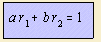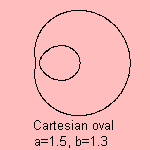# Cartesian oval

## quartic

last updated: 2004-12-04with foci F1 (-1, 0) and F2 (1,0).
This bipolar equation defines the Cartesian oval as the collection of points for which the distances to F1 and F2 are related linearly.The curve is also called the oval of Descartes, or the Cartesian curve 1).
One can imagine that

The curve is a quartic, in fact a bicircular quartic and a cyclic of a circle.
When working out the bipolar equation into the Cartesian form 2), a second oval appears. In fact the bipolar equation extends to a r1 ± b r2 = ±1.

When the inside and outside of an Cartesian oval have refraction indices n1 and n2, respectively, with n1/n2 = b/a, then the refracted rays sent from one focal point, seem to be issued from the other focal point. This led to the name of aplanatic curve 3).
For certain values the inner part of the curve has the form of an egg, the egg of Descartes.

Some special cases of the Cartesian oval are:

It was Descartes (1637) who was the first to describe the curve; Newton studied the curve while classifying his cubic curves.

notes

1) In French: cartésienne
In Italian: ovale Cartesiana

2) Cartesian equation of the curve is, with c = a2 - b2 and d = a2 + b2:
(c(x2 +y2+1)-2dx))2 = 2d(x2+y2+1)-4cx-1

This is said to be equivalent with the following:
(x2+y2)2 + k(x2+y2) + lx + m = 0

3) in French: courbe aplanétique